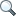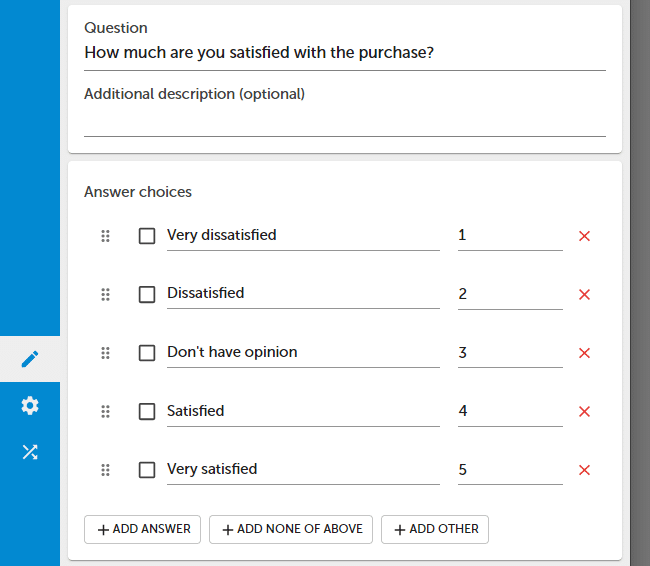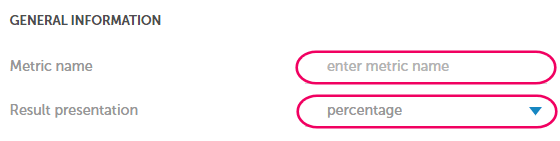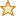SearchHelp sections

# Metrics

Metrics allow you to perform automatic calculations that are based on survey questions and collected responses. You can also use them to set automatic actions eg. send an email or trigger a webhook.

You can use metrics to :

• Create complex indicators like CSI (Customer Satisfaction Index) or CSAT (Customer Satisfaction Intex).
• Calculate the average or sum for the selected group of questions.
• Calculate NPS.
• Create online tests.In order to add a new metric :

1. Add points for each question that will be used to calculate a metric.2. Save changes in the question.
3. Press Metrics button.
4. Press + ADD METRIC button.5. Enter a metric name and select data presentation format.6. Add questions that will be used for calculation or press + ADD ALL QUESTIONS button.7. Metric will be saved automatically.

How points and percentages work:

Type Formula Description
Points ∑ (Q1 + Q2  + ... + Qn) The system will automatically sum up number of points achieved by the respondent.
Points average ∑ (Q1 + Q2  + ... + Qn) / max (Q1 + Q2  + ... + Qn) The system will automatically sum up number of points achieved by the respondent and divide it by the maximum number of points that can be achieved.
Percentage ∑ (Q1 + Q2 +  ... + Qn) / max (Q1 + Q2  + ... + Qn) * 100% The system will automatically sum up number of points achieved by the respondent and divide it by a maximum number of points that can be achieved and present it as a percentage.
NPS %Detractors - %Promotors The system will automatically calculate NPS score. Works only with NPS questions.

Negative values are not used to calculate the maximum score.

Metrics are processed in real-time, just like your survey and reports.

#### Actions

You can set different actions for your metric. Actions will be executed if the metric value will be lower or higher than a defined number of points

• Send email (to a defined email address)
• Create Slack entry
• Create Zendesk ticket
• Create Webhook

Metrics work for :

• Choice questions.
• Star rating questions.
• NPS.
• Matrix (excluding multiple choice matrix question - list).
• Numeric or slider.

NOTE. Metrics are based on points for answer choices. To use this functionality you need to add points for each answer choice.Functionality available in the Advanced, Professional and Enterprise plan.

Related pages